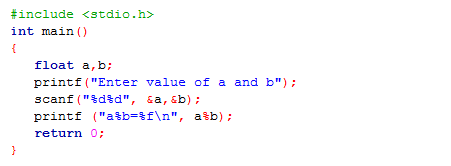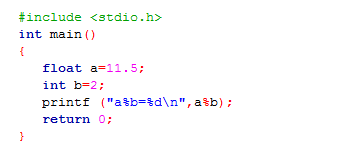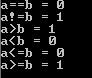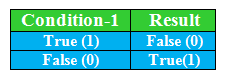## Saturday, 29 December 2018

### OPERATOR IN THE C PROGRAMMING LANGUAGE

Before start deep discussion on the operator. First of all, I would like to do the discussion on three terms which are operator, operand, and operation. OK, anyone tells me what is it? No, OK fine, I will explain to you. Start with the term operation, the operation is nothing it is just a process. Understand the meaning of this line, no, it's OK, No issue. Try to understand operation with the lock and a key example I want to open the lock using a key, in this line what is the process is going to happen that is opening a lock right. The task or action which you performed is called operation. Now, anybody can tell me what is operand from the lock and key example, of course, lock and key are operands, quantity or thing on which operation is going to be performed is called operand. Now, come to our main term i.e. the operator when a person derives or operate a machine or equipment or device is called operator, simple is that in mathematics '+' is the operator which perform the addition operation on the operand.

Operator in the C programming language is a symbol which tells the compiler which operation is going to be performed or which operation is to be performed on the operand.

### Recommended Posts

What is Array in C?

For example

g=10, f=29

output= g+f;

In the above example, g and f are operands, '+' is operator and addition is an operation.Operators in C

### TYPES OF OPERATOR IN C

#### Arithmetic operator

The arithmetic operator performs the mathematical operations like addition, subtraction, multiplication, division and modulus operation.Symbols of Arithmetic Operator

• C Program to Demonstrate the Working of OperatorC Program to Demonstrate the Working of Arithmetic Operator

When you compile and  run above program in Turbo C or in Code Block You will get the following result:

Enter  the value of a and b 12 3

a+b=15
a-b=9
a*b=36
a/b=4
a%b=0

The calculation of addition, subtraction, multiplication, division and modulus is similar to the mathematical calculation. Modulus operator is used to getting the remainder because the value of variable a and b is integer type and is completely divisible so we get remainder 0. Let's find out what happened:
1. When both values i.e. 'a' and 'b' are float data type
2. When 'a' is float data type and 'b' is an int data type.

1. When both values i.e. 'a' and 'b' are float data typeOperation of Modulus operator when both types of data are float

Output

error: invalid operands to binary % (have 'float'  and 'float')

An error occurs after compilation of the above program because modulus operator is not applicable when both the operands are of the float data type.

2. When 'a' is float data type and 'b' is an int data type.Modulus operator when one type of data is a float and other is int

Output

error: invalid operands to binary % (have 'float' and 'int')

An error occurs after compilation of the above program because modulus operator is only worked on an integer data type values.

• If you want to print two digits after decimal point then instead of writing %f you will write %0.2f and now only two most significant bits are printed after a decimal point or if you want to print four digits after decimal point then you can write %0.4f.

For example

#include <stdio.h>
int main ( )
{
float a; int b;
printf ("Enter value of a and b");
scanf ("%d%d", &a,&b);
printf ("a/b=%f", a/b);
printf ("a/b=%0.2f", a/b);
printf ("a/b=%0.4f", a/b);
return 0;
}

Output

Enter the value of a and b 11 2
a/b=5.500000
a/b=5.50
a/b=5.5000

#### 2. Unary Operator

The unary operator operates on a single operand that means it only needs one variable to perform an operation.

• Unary minus: Symbol of unary minus is denoted as (-). Unary minus is different from a subtraction operator because subtraction operator needs two operands to operate but unary minus needs only one operand to operate. Unary minus is used to make positive value negative or vice versa.

For example

int g = 67;
int f = - g     // f = - 67

OR
int  g= -67
int f = -g;      // f=67

• Increment operator: Increment operator is used to incrementing the value of operand by one. Increment operator is denoted by the symbol (++).

Post-increment: This increment first used the value then increases the value of an operand.

For example

int x= 10;
printf ("x++=%d\n\n",x++);

First of all value of x is printed i.e. x=10 then the value of x is incremented by one i.e x++=11.

printf ("value of x after post-increment of x=%d\n\n", x);        // Output: x=11

Pre-increment: In pre-increment, the first value of the operand is incremented by one then it is used further.

For example:

int z = 34;
printf ("++z=%d\n", ++z);

Firstly, the value of z is incremented by one i.e. ++z=35 then the value of z is printed.

• Decrement operator: Decrement operator is used to decrementing the value of operand by one. Decrement operator is denoted by the symbol (--).

Post-decrement: This decrement first used the value then decreases the value of an operand by one.

For example

int w = 45;
printf ("w=%d\n", w--);

Firstly, the value of w is printed i.e w= 45 then it is decreased by one i.e. w = 44.

Pre-decrement: In pre-decrement, the first value of the operand is decremented by one then it is used further.

For example

int w = 45;
printf ("w=%d\n", --w);

First of all, the value of w is decremented by one i.e. w=44 then it is printed.

• Logical Not operator: Logical Not operator is denoted as (!) and it reverses the logical state of the operand.

For example

if the value of k is true, then !k is false
if the value of k is false, then !k is true

• sizeof ( ) operator: sizeof operator is used to calculating the size of the operand.

For example

int a;
sizeof (a);

After the run of "sizeof" instruction compiler tells the size of integer 'a' i.e 4 bytes. Similarly, if you want to find out the size of float, double, char etc. you can write "sizeof" command before operand.

#### 3. Assignment Operator

The assignment operator is used to assign a value to an operand. Associativity of the assignment operator is from right to left i.e. value give on the right-hand side of an operator is assigned to the variable on the left.

Variable = Value;

The assignment operator is combined with other operators to make a shorter version like

a = a+15 can be written as a +=15. This operator first adds the 15 to the value of 'a' and then transfer to operand 'a'.
Similarly, a = a-15 can be written as a-=15.
a = a/15, a = a*15 and a = a%15 can be written as a/=15, a*=15 and a%=15.

#### 4. Relational Operator

Relational operator is used to checks the relation between two variable like a comparison, greater than, less than etc. If that relation or expression is true then it gives/returns true value i.e. 1 otherwise zero/ false value.

== equal to operator checks the value of two variables if the value of operands is equal then it returns true value i.e. one otherwise false i.e. zero.

!= not equal to operator is used to checks the value of the given operand is equal or not, if it is equal it returns false value else true value i.e. 1.

> greater than operator checks the value of the first operand is greater than the second operand if it is true then it return 1 else it returns 0.

< less than operator checks the value of the first operand is less than the second operand if yes then it returns 1  else it returns zero.

<= less than equal to operator checks the value of the first operand is less than or equal to the second operand if yes then it returns 1 otherwise it returns zero.

>= greater than equal to operator checks the value of the first operand is greater than or equal to the second operand if yes then it returns 1 else it returns zero.

• C program to demonstrate the working of the relational operators.Example of working of Relational Operator

OutputAn output of the above program

#### 5. Logical Operator

Logical operators are used to performing a logical operation like AND, OR and NOT operation. The operation of these operators are similar to the operation of AND, OR and NOT gate in digital electronics. A logical operator is used to checking two or more conditions. Logical operators are described below:

• Logical AND operator: When both conditions are true (any non-zero value is considered as true value) then the output is true if any single condition is false then the output is false. Logical AND operator is denoted by the symbol (&&).Table of Logical AND operator

For example

int b = 19;

(b 20) && (b++)

First of all, the compiler checks the value of 'b' which is greater than 20 or not? If it's not, then compiler not move to check next condition and gives false output. If the value of 'b' is greater than 20 then the compiler will check the condition i.e. increase the value of 'b' by one. Because 'b > 20' and ' b++' is true then output will be true.

• Logical OR operator: Any single condition is true (any non-zero value) then the output will be true. Logical OR operator is denoted as (||).Table of Logical OR operator

For example

int w = 20;
(w > 21) || (w == 20)

In the above expression, first checks the condition (w > 21) or not, the value of the operand w is 20 and the condition w > 21 becomes false because 20 is not greater than 21. Now the compiler checks the next condition mention in the expression i.e. w==20 and this condition is true. So, the output of the expression is true.

• Logical NOT operator: This operator is a unary operator which reverses the value i.e. if the value is true then this operator make the value false or vice-versa. A symbol used for NOT operator is (!).Table of Logical NOT operator

#### 6. Ternary Operator

Ternary means three objects whenever any operator operates on three operands are known as Ternary operator. The ternary operator is a shorter version of the if-else statement. It is also known as a conditional operator.

Syntax

Condition-1 ? statement-2 : statement-3

The above statement says that when condition-1 is true then the statement-2 is operated else statement-3 is operated.Ternary operator

In the above program, when condition (a > =b) is evaluated to true then the output is k=c else k= d.

Output

The final result of k = 20

#### 7. Bitwise operator

These operators are used to perform manipulation of single bits of a number. This can be used with any of the integer types. Bitwise operators are shown belowDescription of Bitwise Operator

• A program which shows the working of all Bitwise OperatorBitwise Operator

OutputAn output of the Bitwise Operator

• Left Shift Operator (<<): This operator shifts the bits of a number to the left and put zero to the right side.
For example

0000 1111 << 2 will shift the two bits of a number to the left then the output will be 0011 1100.

Try to understand it using one more example  1111 0011<< 2 and the output is 1100 1100.

• Right Shift Operator (>>): This operator shifts the bits of a number to the right and put zero to left as a result.

For example

0000 1111 >>2 will shift the two bits of a number to the right then the output will be 0011 1100.

#### 1 comment:

1.